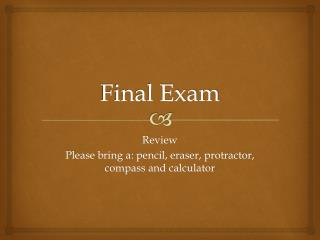DownloadDownload PresentationFinal Exam

# Final Exam

Download Presentation## Final Exam

- - - - - - - - - - - - - - - - - - - - - - - - - - - E N D - - - - - - - - - - - - - - - - - - - - - - - - - - -
##### Presentation Transcript

1. Final Exam Review Please bring a: pencil, eraser, protractor, compass and calculator

2. Chapter 1 • A number is divisible by 2, if it is an even number (ends in 0,2,4,6,8). • A number is divisible by 3, if the sum of the digits is divisible by 3. • A number is divisible by 4, if the last two digits are divisible by 4. • A number is divisible by 5, if it ends in a O or a 5. • A number is divisible by 6, if it is divisible by 2 and 3. • A number is divisible by 8, if the last three digits are divisible by 8. • A number is divisible by 9, if the sum of the digits is divisible by 9. • A number is divisible by 10, if the last digit is a 0.

3. Chapter 1

4. Chapter 1

5. Chapter 1- practice

6. Answers 2 9, 18, 27, 36, 45

7. Chapter 1 • Extra practice: • Page 37-38

8. Final Exam Review Chapter 2

9. Chapter 2

10. Chapter 2- practice

11. Chapter 2- practice 3/14 8, 16

12. Chapter 2

13. Chapter 2-practice 4 5/8 2 4/5 4 19/20 7 6/11

14. Chapter 2

15. Chapter 2- practice 21/9 57/10

16. Chapter 2

17. Chapter 2- practice 2/8 Or ¼ 5/6

18. Chapter 2

19. Chapter 2- practice 45/8 Or 5 5/8 29/12 Or 2 5/12 5/4

20. Chapter 2 • Extra practice • Page 89-90 • Page 93-94

21. Final Exam Review Chapter 3

22. Chapter 3

23. Chapter 3- practice 1.356 + 0.405 + 22.015= 2.5 + 12.6 + 20.9= 4.123 – 3.200= 9.623-5.061=

24. Practice - answers 1.356 + 0.405 + 22.015= 23.776 2.5 + 12.6 + 20.9= 36 4.123 – 3.200= 0.923 9.623-5.061= 4.562

25. Chapter 3

26. Chapter 3- practice • 3.4 x 0.2= • 7.6 x 0.8= • 3.67 x 1.01= • 10.19 x 0.35=

27. Practice- Answers • 3.4 x 0.2= 0.68 • 7.6 x 0.8= 6.08 • 3.67 x 1.01= 3.7067 • 10.19 x 0.35= 3.5665

28. Chapter 3

29. Chapter 3- practice • 3.6 0.18= • 9.72 2.7= • 4.59 0.9= • 3.13 3.13=

30. Practice - Answers • 3.6 0.18= 20 • 9.72 2.7= 3.6 • 4.59 0.9= 5.1 • 3.13 3.13= 1

31. Chapter 3

32. Chapter 3- practice • 32.28- (1.2 • 10.9 + (3.3 x3.3- 1.69)

33. Practice- Answers • 32.28- (1.2= 28.78 • 10.9 + (3.3 x3.3- 1.69) = 8.77

34. Chapter 3 • Extra Practice: • Page 143-144 • Page 145-146

35. Final Exam Review Chapter 4

36. Chapter 4

37. Chapter 4- practice

38. Practice- Answers 60/100 = 3/5 0.60 9% 9/100 0.03 3% 44/100= 11/25 0.44 24% 0.24 50% 50/100 = 1/2 1.00 100% 12/100= 3/25 0.12

39. Chapter 4- practice

40. Practice- Answers 30 9 110 70 16 7 x100 Then divide by 10

41. Chapter 4 • Extra practice • Page 185 • Page 187-188

42. Final Exam Review Chapter 5

43. Chapter 5

44. Chapter 5- practice A= 15

45. Chapter 5- practice A= 71.5 A= 6

46. Chapter 5- practice C= 32 cm C= 57.78

47. Chapter 5- practice 7.07 289.4

48. Chapter 5- practice A rectangle= 3.19 A circle= 3.30 Total= 6.49

49. Chapter 5 • Extra practice • Page 231-232 • Page 233-234

50. Final Exam Review Chapter 6